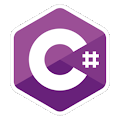Programming Course in C# ¡Free!

# Array of objects : table

Proposed exercise

Create a class named "Table". It must have a constructor, indicating the width and height of the board. It will have a method "ShowData" which will write on the screen the width and that height of the table. Create an array containing 10 tables, with random sizes between 50 and 200 cm, and display all the data.

Output

Solution

```using System;
namespace ArrayOfObjects
{
class Table
{
private float width, height;

public Table()
{
}
public Table(float width, float height)
{
this.width = width;
this.height = height;
}

public float Width
{
set { width = value; }
get { return width; }
}
public float Height
{
set { height = value; }
get { return height; }
}

public void ShowData()
{
Console.WriteLine("Width: {0}, Heigth: {1}",width,height);
}
}

class TestTables
{
static void Main()
{
bool debug = false;

Table[] myTables = new Table;
Random rnd = new Random();

for (int i = 0; i < 10; i++)
{
myTables[i] = new Table(rnd.Next(50, 201), rnd.Next(50, 201));
myTables[i].ShowData();
}

if (debug)